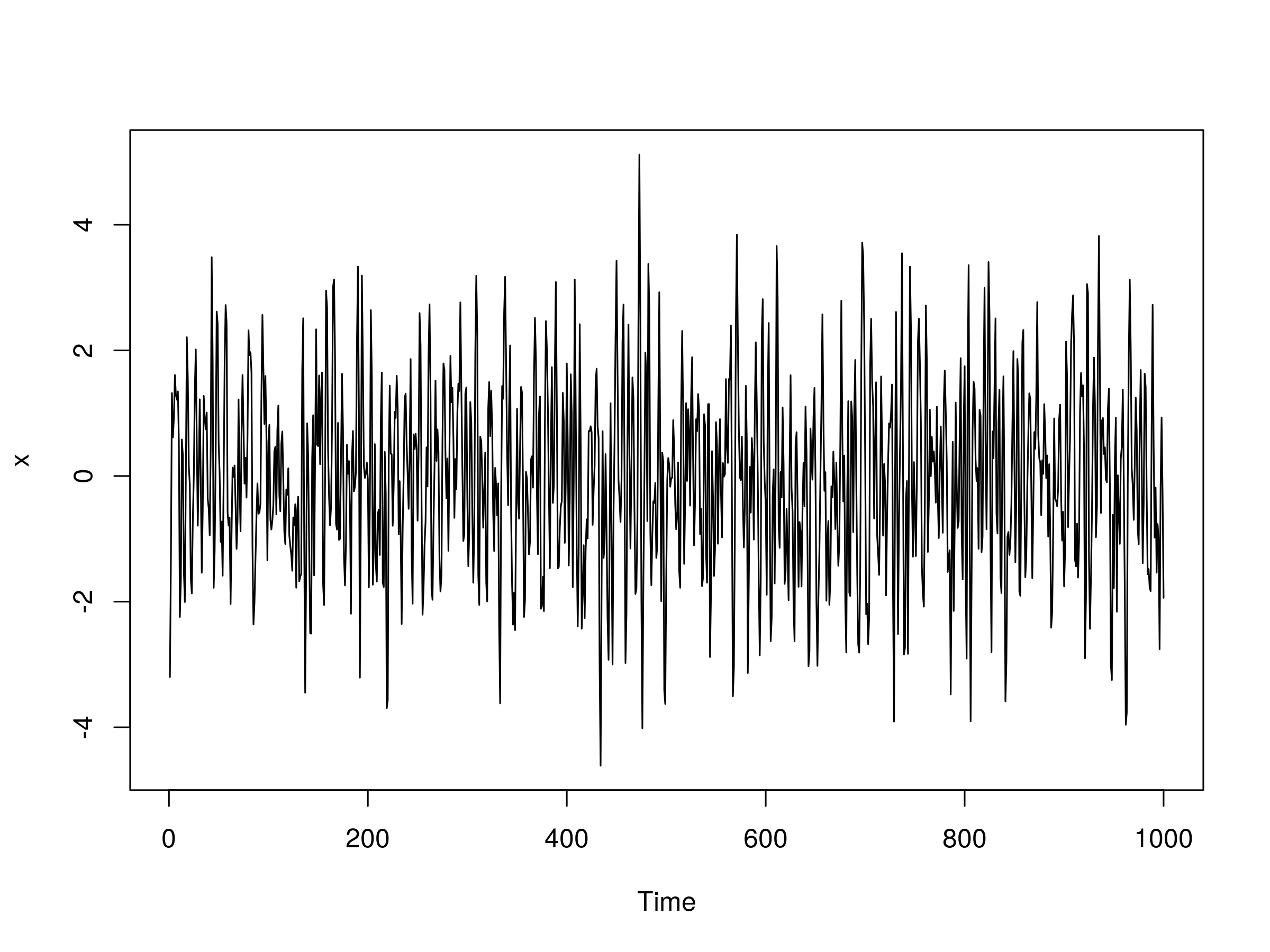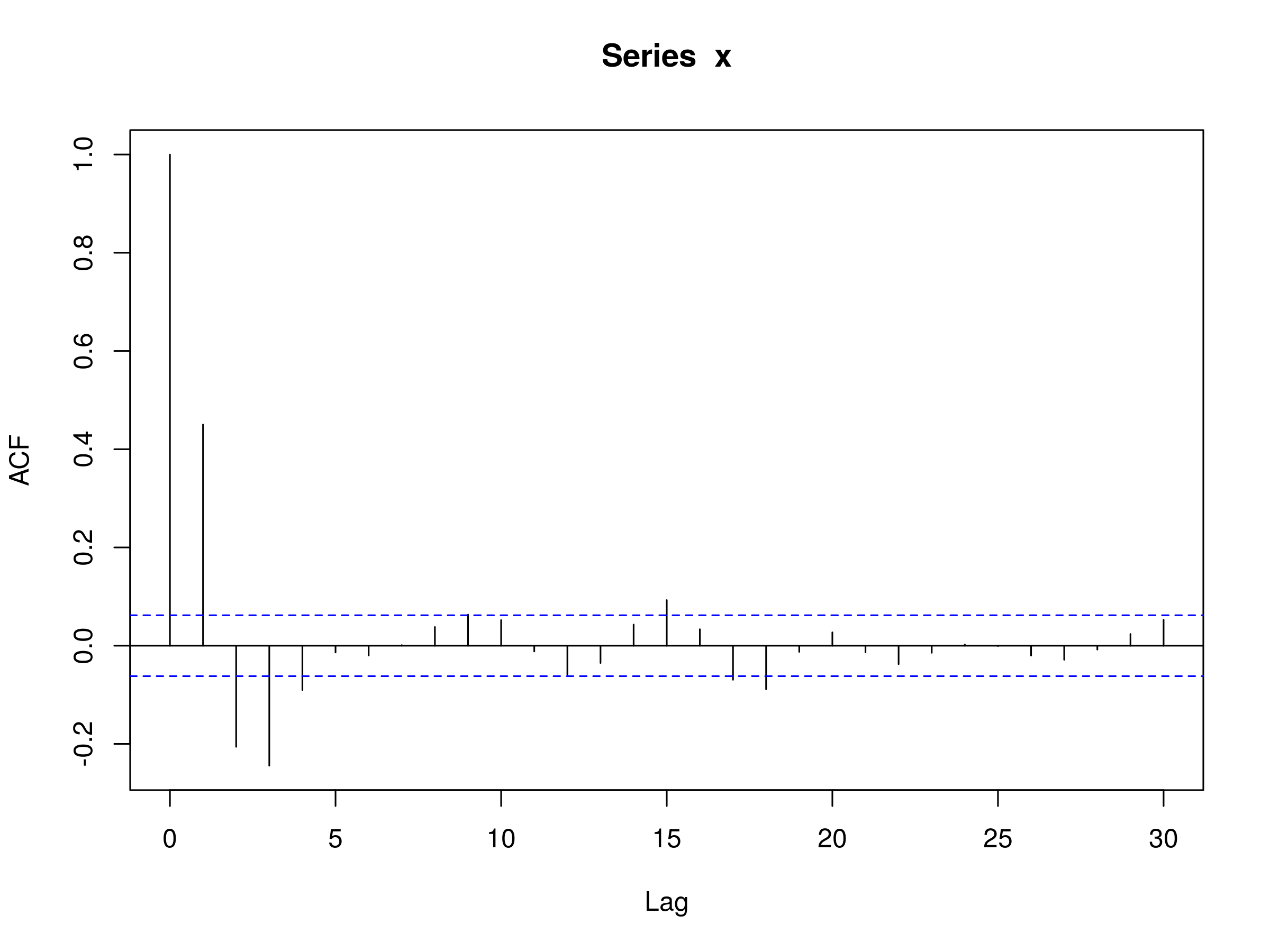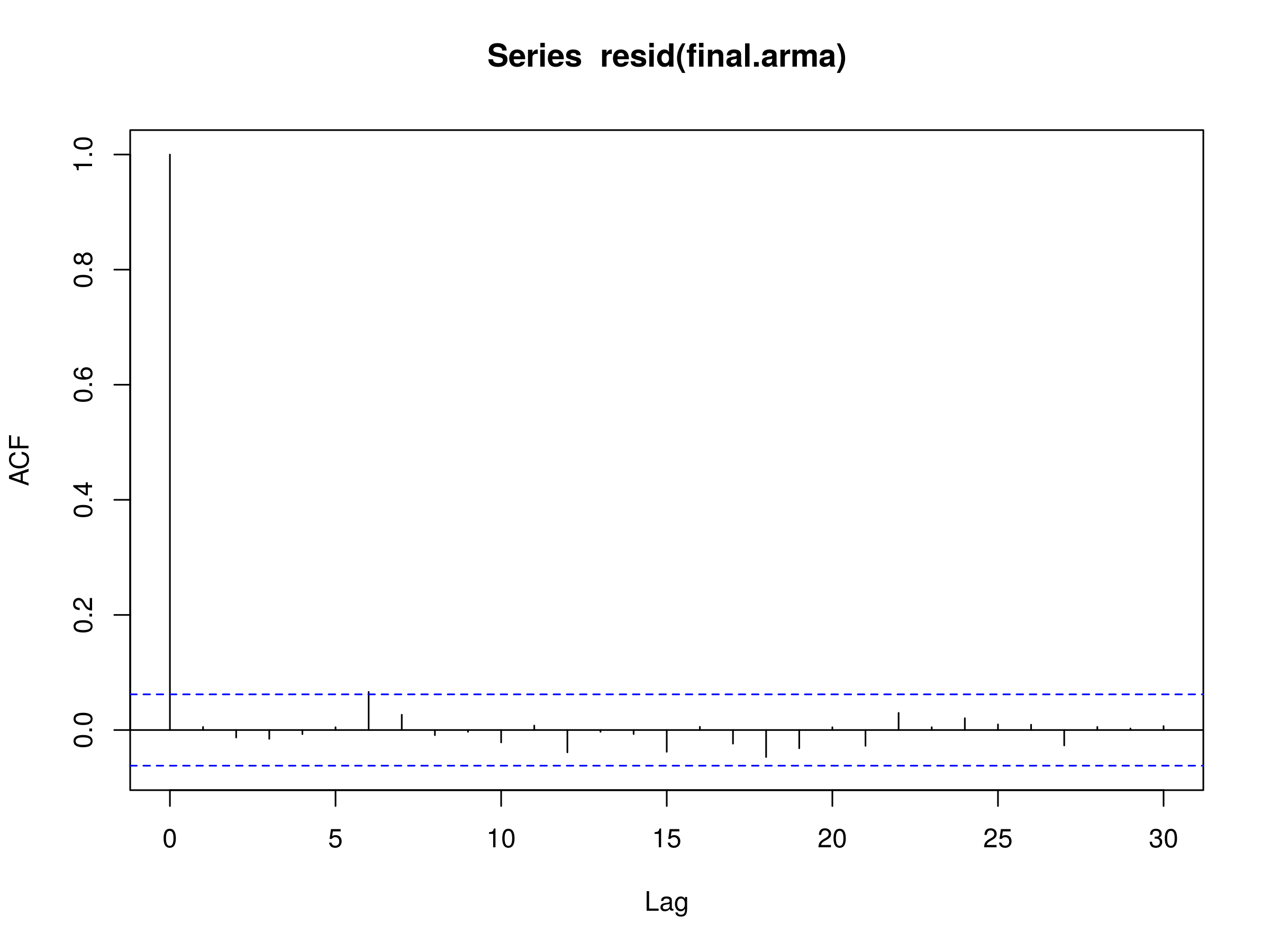# Autoregressive Moving Average ARMA(p, q) Models for Time Series Analysis - Part 3

Autoregressive Moving Average ARMA(p, q) Models for Time Series Analysis - Part 3

This is the third and final post in the mini-series on Autoregressive Moving Average (ARMA) models for time series analysis. We've introduced Autoregressive models and Moving Average models in the two previous articles. Now it is time to combine them to produce a more sophisticated model.

Ultimately this will lead us to the ARIMA and GARCH models that will allow us to predict asset returns and forecast volatility. These models will form the basis for trading signals and risk management techniques.

If you've read Part 1 and Part 2 you will have seen that we tend to follow a pattern for our analysis of a time series model. I'll repeat it briefly here:

• Rationale - Why are we interested in this particular model?
• Definition - A mathematical definition to reduce ambiguity.
• Correlogram - Plotting a sample correlogram to visualise a models behaviour.
• Simulation and Fitting - Fitting the model to simulations, in order to ensure we've understood the model correctly.
• Real Financial Data - Apply the model to real historical asset prices.
• Prediction - Forecast subsequent values to build trading signals or filters.

In order to follow this article it is advisable to take a look at the prior articles on time series analysis. They can all be found here.

## Bayesian Information Criterion

In Part 1 of this article series we looked at the Akaike Information Criterion (AIC) as a means of helping us choose between separate "best" time series models.

A closely related tool is the Bayesian Information Criterion (BIC). Essentially it has similar behaviour to the AIC in that it penalises models for having too many parameters. This may lead to overfitting. The difference between the BIC and AIC is that the BIC is more stringent with its penalisation of additional parameters.

#### Bayesian Information Criterion

If we take the likelihood function for a statistical model, which has $k$ parameters, and $L$ maximises the likelihood, then the Bayesian Information Criterion is given by:

\begin{eqnarray} BIC = -2 \text{log} (L) + k \text{log}(n) \end{eqnarray}

Where $n$ is the number of data points in the time series.

We will be using the AIC and BIC below when choosing appropriate ARMA(p,q) models.

## Ljung-Box Test

In Part 1 of this article series Rajan mentioned in the Disqus comments that the Ljung-Box test was more appropriate than using the Akaike Information Criterion of the Bayesian Information Criterion in deciding whether an ARMA model was a good fit to a time series.

The Ljung-Box test is a classical hypothesis test that is designed to test whether a set of autocorrelations of a fitted time series model differ significantly from zero. The test does not test each individual lag for randomness, but rather tests the randomness over a group of lags.

Formally:

#### Ljung-Box Test

We define the null hypothesis ${\bf H_0}$ as: The time series data at each lag are i.i.d., that is, the correlations between the population series values are zero.

We define the alternate hypothesis ${\bf H_a}$ as: The time series data are not i.i.d. and possess serial correlation.

We calculate the following test statistic, $Q$:

\begin{eqnarray} Q = n (n+2) \sum_{k=1}^{h} \frac{\hat{\rho}^2_k}{n-k} \end{eqnarray}

Where $n$ is the length of the time series sample, $\hat{\rho}_k$ is the sample autocorrelation at lag $k$ and $h$ is the number of lags under the test.

The decision rule as to whether to reject the null hypothesis ${\bf H_0}$ is to check whether $Q > \chi^2_{\alpha,h}$, for a chi-squared distribution with $h$ degrees of freedom at the $100(1-\alpha)$th percentile.

While the details of the test may seem slightly complex, we can in fact use R to calculate the test for us, simplifying the procedure somewhat.

## Autogressive Moving Average (ARMA) Models of order p, q

Now that we've discussed the BIC and the Ljung-Box test, we're ready to discuss our first mixed model, namely the Autoregressive Moving Average of order p, q, or ARMA(p,q).

### Rationale

To date we have considered autoregressive processes and moving average processes.

The former model considers its own past behaviour as inputs for the model and as such attempts to capture market participant effects, such as momentum and mean-reversion in stock trading.

The latter model is used to characterise "shock" information to a series, such as a surprise earnings announcement or unexpected event (such as the BP Deepwater Horizon oil spill).

Hence, an ARMA model attempts to capture both of these aspects when modelling financial time series.

Note that an ARMA model does not take into account volatility clustering, a key empirical phenomena of many financial time series. It is not a conditionally heteroscedastic model. For that we will need to wait for the ARCH and GARCH models.

### Definition

The ARMA(p,q) model is a linear combination of two linear models and thus is itself still linear:

#### Autoregressive Moving Average Model of order p, q

A time series model, $\{ x_t \}$, is an autoregressive moving average model of order $p,q$, ARMA(p,q), if:

\begin{eqnarray} x_t = \alpha_1 x_{t-1} + \alpha_2 x_{t-2} + \ldots + w_t + \beta_1 w_{t-1} + \beta_2 w_{t-2} \ldots + \beta_q w_{t-q} \end{eqnarray}

Where $\{ w_t \}$ is white noise with $E(w_t) = 0$ and variance $\sigma^2$.

If we consider the Backward Shift Operator, ${\bf B}$ (see a previous article) then we can rewrite the above as a function $\theta$ and $\phi$ of ${\bf B}$:

\begin{eqnarray} \theta_p ({\bf B}) x_t = \phi_q ({\bf B}) w_t \end{eqnarray}

We can straightforwardly see that by setting $p \neq 0$ and $q=0$ we recover the AR(p) model. Similarly if we set $p = 0$ and $q \neq 0$ we recover the MA(q) model.

One of the key features of the ARMA model is that it is parsimonious and redundant in its parameters. That is, an ARMA model will often require fewer parameters than an AR(p) or MA(q) model alone. In addition if we rewrite the equation in terms of the BSO, then the $\theta$ and $\phi$ polynomials can sometimes share a common factor, thus leading to a simpler model.

### Simulations and Correlograms

As with the autoregressive and moving average models we will now simulate various ARMA series and then attempt to fit ARMA models to these realisations. We carry this out because we want to ensure that we understand the fitting procedure, including how to calculate confidence intervals for the models, as well as ensure that the procedure does actually recover reasonable estimates for the original ARMA parameters.

In Part 1 and Part 2 we manually constructed the AR and MA series by drawing $N$ samples from a normal distribution and then crafting the specific time series model using lags of these samples.

However, there is a more straightforward way to simulate AR, MA, ARMA and even ARIMA data, simply by using the arima.sim method in R.

Let's start with the simplest possible non-trivial ARMA model, namely the ARMA(1,1) model. That is, an autoregressive model of order one combined with a moving average model of order one. Such a model has only two coefficients, $\alpha$ and $\beta$, which represent the first lags of the time series itself and the "shock" white noise terms. Such a model is given by:

\begin{eqnarray} x_t = \alpha x_{t-1} + w_t + \beta w_{t-1} \end{eqnarray}

We need to specify the coefficients prior to simulation. Let's take $\alpha = 0.5$ and $\beta = -0.5$:

> set.seed(1)
> x <- arima.sim(n=1000, model=list(ar=0.5, ma=-0.5))
> plot(x)


The output is as follows:Realisation of an ARMA(1,1) Model, with $\alpha=0.5$ and $\beta = 0.5$

Let's also plot the correlogram:

> acf(x)Correlogram of an ARMA(1,1) Model, with $\alpha=0.5$ and $\beta = 0.5$

We can see that there is no significant autocorrelation, which is to be expected from an ARMA(1,1) model.

Finally, let's try and determine the coefficients and their standard errors using the arima function:

> arima(x, order=c(1, 0, 1))

Call:
arima(x = x, order = c(1, 0, 1))

Coefficients:
ar1     ma1  intercept
-0.3957  0.4503     0.0538
s.e.   0.3727  0.3617     0.0337

sigma^2 estimated as 1.053:  log likelihood = -1444.79,  aic = 2897.58


We can calculate the confidence intervals for each parameter using the standard errors:

> -0.396 + c(-1.96, 1.96)*0.373
 -1.12708  0.33508
> 0.450 + c(-1.96, 1.96)*0.362
 -0.25952  1.15952


The confidence intervals do contain the true parameter values for both cases, however we should note that the 95% confidence intervals are very wide (a consequence of the reasonably large standard errors).

Let's now try an ARMA(2,2) model. That is, an AR(2) model combined with a MA(2) model. We need to specify four parameters for this model: $\alpha_1$, $\alpha_2$, $\beta_1$ and $\beta_2$. Let's take $\alpha_1 = 0.5$, $\alpha_2=-0.25$ $\beta_1=0.5$ and $\beta_2=-0.3$:

> set.seed(1)
> x <- arima.sim(n=1000, model=list(ar=c(0.5, -0.25), ma=c(0.5, -0.3)))
> plot(x)


The output of our ARMA(2,2) model is as follows:Realisation of an ARMA(2,2) Model, with $\alpha_1=0.5$, $\alpha_2=-0.25$, $\beta_1=0.5$ and $\beta_2 = -0.3$

And the corresponding autocorelation:

> acf(x)Correlogram of an ARMA(2,2) Model, with $\alpha_1=0.5$, $\alpha_2=-0.25$, $\beta_1=0.5$ and $\beta_2 = -0.3$

We can now try fitting an ARMA(2,2) model to the data:

> arima(x, order=c(2, 0, 2))

Call:
arima(x = x, order = c(2, 0, 2))

Coefficients:
ar1      ar2     ma1      ma2  intercept
0.6529  -0.2291  0.3191  -0.5522    -0.0290
s.e.  0.0802   0.0346  0.0792   0.0771     0.0434

sigma^2 estimated as 1.06:  log likelihood = -1449.16,  aic = 2910.32


We can also calculate the confidence intervals for each parameter:

> 0.653 + c(-1.96, 1.96)*0.0802
 0.495808 0.810192
> -0.229 + c(-1.96, 1.96)*0.0346
 -0.296816 -0.161184
> 0.319 + c(-1.96, 1.96)*0.0792
 0.163768 0.474232
> -0.552 + c(-1.96, 1.96)*0.0771
 -0.703116 -0.400884


Notice that the confidence intervals for the coefficients for the moving average component ($\beta_1$ and $\beta_2$) do not actually contain the original parameter value. This outlines the danger of attempting to fit models to data, even when we know the true parameter values!

However, for trading purposes we just need to have a predictive power that exceeds chance and produces enough profit above transaction costs, in order to be profitable in the long run.

Now that we've seen some examples of simulated ARMA models we need mechanism for choosing the values of $p$ and $q$ when fitting to the models to real financial data.

### Choosing the Best ARMA(p,q) Model

In order to determine which order $p,q$ of the ARMA model is appropriate for a series, we need to use the AIC (or BIC) across a subset of values for $p,q$, and then apply the Ljung-Box test to determine if a good fit has been achieved, for particular values of $p,q$.

To show this method we are going to firstly simulate a particular ARMA(p,q) process. We will then loop over all pairwise values of $p \in \{ 0,1,2,3,4 \}$ and $q \in \{ 0,1,2,3,4 \}$ and calculate the AIC. We will select the model with the lowest AIC and then run a Ljung-Box test on the residuals to determine if we have achieved a good fit.

Let's begin by simulating an ARMA(3,2) series:

> set.seed(3)
> x <- arima.sim(n=1000, model=list(ar=c(0.5, -0.25, 0.4), ma=c(0.5, -0.3)))


We will now create an object final to store the best model fit and lowest AIC value. We loop over the various $p,q$ combinations and use the current object to store the fit of an ARMA(i,j) model, for the looping variables $i$ and $j$.

If the current AIC is less than any previously calculated AIC we set the final AIC to this current value and select that order. Upon termination of the loop we have the order of the ARMA model stored in final.order and the ARIMA(p,d,q) fit itself (with the "Integrated" $d$ component set to 0) stored as final.arma:

> final.aic <- Inf
> final.order <- c(0,0,0)
> for (i in 0:4) for (j in 0:4) {
>   current.aic <- AIC(arima(x, order=c(i, 0, j)))
>   if (current.aic < final.aic) {
>     final.aic <- current.aic
>     final.order <- c(i, 0, j)
>     final.arma <- arima(x, order=final.order)
>   }
> }


Let's output the AIC, order and ARIMA coefficients:

> final.aic
 2863.365

> final.order
 3 0 2

> final.arma

Call:
arima(x = x, order = final.order)

Coefficients:
ar1      ar2     ar3     ma1      ma2  intercept
0.4470  -0.2822  0.4079  0.5519  -0.2367     0.0274
s.e.  0.0867   0.0345  0.0309  0.0954   0.0905     0.0975

sigma^2 estimated as 1.009:  log likelihood = -1424.68,  aic = 2863.36


We can see that the original order of the simulated ARMA model was recovered, namely with $p=3$ and $q=2$. We can plot the corelogram of the residuals of the model to see if they look like a realisation of discrete white noise (DWN):

> acf(resid(final.arma))Correlogram of the residuals of the best fitting ARMA(p,q) Model, $p=3$ and $q=2$

The corelogram does indeed look like a realisation of DWN. Finally, we perform the Ljung-Box test for 20 lags to confirm this:

> Box.test(resid(final.arma), lag=20, type="Ljung-Box")

Box-Ljung test

data:  resid(final.arma)
X-squared = 13.1927, df = 20, p-value = 0.869


Notice that the p-value is greater than 0.05, which states that the residuals are independent at the 95% level and thus an ARMA(3,2) model provides a good model fit.

Clearly this should be the case since we've simulated the data ourselves! However, this is precisely the procedure we will use when we come to fit ARMA(p,q) models to the S&P500 index in the following section.

### Financial Data

Now that we've outlined the procedure for choosing the optimal time series model for a simulated series, it is rather straightforward to apply it to financial data. For this example we are going to once again choose the S&P500 US Equity Index.

Let's download the daily closing prices using quantmod and then create the log returns stream:

> require(quantmod)
> getSymbols("^GSPC")
> sp = diff(log(Cl(GSPC)))


Let's perform the same fitting procedure as for the simulated ARMA(3,2) series above on the log returns series of the S&P500 using the AIC:

> spfinal.aic <- Inf
> spfinal.order <- c(0,0,0)
> for (i in 0:4) for (j in 0:4) {
>   spcurrent.aic <- AIC(arima(sp, order=c(i, 0, j)))
>   if (spcurrent.aic < spfinal.aic) {
>     spfinal.aic <- spcurrent.aic
>     spfinal.order <- c(i, 0, j)
>     spfinal.arma <- arima(sp, order=spfinal.order)
>   }
> }


The best fitting model has order ARMA(3,3):

> spfinal.order
 3 0 3


Let's plot the residuals of the fitted model to the S&P500 log daily returns stream:

> acf(resid(spfinal.arma), na.action=na.omit)Correlogram of the residuals of the best fitting ARMA(p,q) Model, $p=3$ and $q=3$, to the S&P500 daily log returns stream

Notice that there are some significant peaks, especially at higher lags. This is indicative of a poor fit. Let's perform a Ljung-Box test to see if we have statistical evidence for this:

> Box.test(resid(spfinal.arma), lag=20, type="Ljung-Box")

Box-Ljung test

data:  resid(spfinal.arma)
X-squared = 37.1912, df = 20, p-value = 0.0111


As we suspected, the p-value is less that 0.05 and as such we cannot say that the residuals are a realisation of discrete white noise. Hence there is additional autocorrelation in the residuals that is not explained by the fitted ARMA(3,3) model.

## Next Steps

As we've discussed all along in this article series we have seen evidence of conditional heteroscedasticity (volatility clustering) in the S&P500 series, especially in the periods around 2007-2008. When we use a GARCH model later in the article series we will see how to eliminate these autocorrelations.

In practice, ARMA models are never generally good fits for log equities returns. We need to take into account the conditional heteroscedasticity and use a combination of ARIMA and GARCH. The next article will consider ARIMA and show how the "Integrated" component differs from the ARMA model we have been considering in this article.